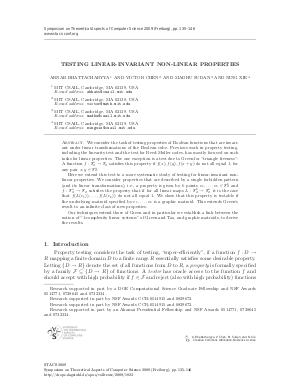Document# Testing Linear-Invariant Non-Linear Properties

### Authors Arnab Bhattacharyya, Victor Chen, Madhu Sudan, Ning Xie## File

LIPIcs.STACS.2009.1823.pdf
• Filesize: 188 kB
• 12 pages

## Cite As

Arnab Bhattacharyya, Victor Chen, Madhu Sudan, and Ning Xie. Testing Linear-Invariant Non-Linear Properties. In 26th International Symposium on Theoretical Aspects of Computer Science. Leibniz International Proceedings in Informatics (LIPIcs), Volume 3, pp. 135-146, Schloss Dagstuhl - Leibniz-Zentrum für Informatik (2009)
https://doi.org/10.4230/LIPIcs.STACS.2009.1823

## Abstract

We consider the task of testing properties of Boolean functions that are invariant under linear transformations of the Boolean cube. Previous work in property testing, including the linearity test and the test for Reed-Muller codes, has mostly focused on such tasks for linear properties. The one exception is a test due to Green for {}triangle freeness'': A function $f:\mathbb{F}_{2}^{n}\to\mathbb{F}_{2}$ satisfies this property if $f(x),f(y),f(x+y)$ do not all equal $1$, for any pair $x,y\in\mathbb{F}_{2}^{n}$. Here we extend this test to a more systematic study of testing for linear-invariant non-linear properties. We consider properties that are described by a single forbidden pattern (and its linear transformations), i.e., a property is given by $k$ points $v_{1},\ldots,v_{k}\in\mathbb{F}_{2}^{k}$ and $f:\mathbb{F}_{2}^{n}\to\mathbb{F}_{2}$ satisfies the property that if for all linear maps $L:\mathbb{F}_{2}^{k}\to\mathbb{F}_{2}^{n}$ it is the case that $f(L(v_{1})),\ldots,f(L(v_{k}))$ do not all equal $1$. We show that this property is testable if the underlying matroid specified by $v_{1},\ldots,v_{k}$ is a graphic matroid. This extends Green's result to an infinite class of new properties. Our techniques extend those of Green and in particular we establish a link between the notion of {}1-complexity linear systems'' of Green and Tao, and graphic matroids, to derive the results.

## Metrics

• Access Statistics
• Total Accesses (updated on a weekly basis)
0